Форум - Замок

# Картинная галерея: Планеты в аспектах и Домах.

## Recommended Posts

• Replies 164
• Created

#### Posted Images

Меркурий в Овне, кличка - Енот :))))))))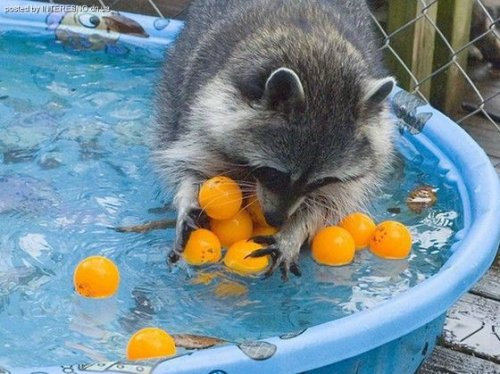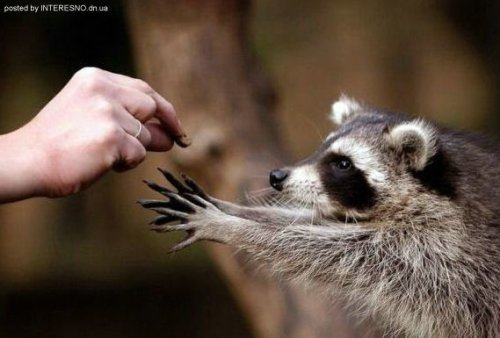)))))))))))))))))))))))))))))))))))))))))))))))

ето уж скорее Юпитер, пораженный реалиями жизни! )))))))))))))

Или - Мерк-ретро в Тельце, или во 2-м доме...))))

классные фотки!

##### Share on other sites

Луна в Раке##### Share on other sites

Луна в Раке - чувствительность, интуиция, психологические способности, ощущение бессознательных реакций других людей, а также фантазерство... Лунный Рак обволакивает теплым облаком сочувствия и понимания...##### Share on other sites

Солнце в соединении с Лилит

Обладатель данного соединения не понЯл...

Можно разъяснить?

##### Share on other sites

Обладатель данного соединения не понЯл...

Можно разъяснить?

Насть, ну для обладателя оного соединения есть в основном одна воля - его собственная, и даже эволюцию он какбы склонен отсчитывать от момента своего рождения.)))

Настя, аспекты - важны, так что...это - мое личное сборное очучение от лилитовцев-солнечных - зацикленные на себе граждане, для которых собственное эго - божественно, теории Дарвина не подверженное...))(я тоже, кстати, в теорию Дарвина как-то не очень..)))

##### Share on other sites

Меркурий в Козероге##### Share on other sites

)))))))))))))))))))))))))))))))))))))))))))))))

ето уж скорее Юпитер, пораженный реалиями жизни! )))))))))))))

Или - Мерк-ретро в Тельце, или во 2-м доме...))))

классные фотки!

А, ну тоже вполне возможно, если Енотский прихватизм брать :))))))

У меня мерк в овне с енотским любопытсвом ассоциируется, так как везде свой нос сунуть надо, Ынтересно и жутко любопытно, причем почти всё, что глаза разбегаются...

##### Share on other sites

Насть, ну для обладателя оного соединения есть в основном одна воля - его собственная, и даже эволюцию он какбы склонен отсчитывать от момента своего рождения.)))

Настя, аспекты - важны, так что...это - мое личное сборное очучение от лилитовцев-солнечных - зацикленные на себе граждане, для которых собственное эго - божественно, теории Дарвина не подверженное...))(я тоже, кстати, в теорию Дарвина как-то не очень..)))

)))

Благо, кроме данного соединения в моей карте присутствуют и другие аспекты...

##### Share on other sites

Уран в оппозиции к Сатурну

Уран слева.))) мне в силу технических возможностей счас ток остается фрагменты из интернов вставалять, благо там - почти все астросочетания наблюдаются..))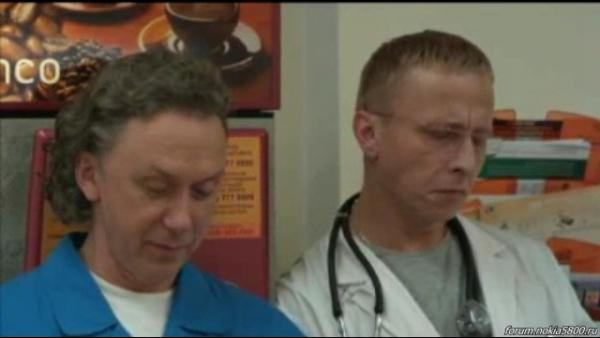##### Share on other sites

Марс во Льве##### Share on other sites

Солнце в Близнецах##### Share on other sites

Солнце в Овне##### Share on other sites

Лилит-квадрат-Луна-квадрат-Сатурн-квадрат-Плутон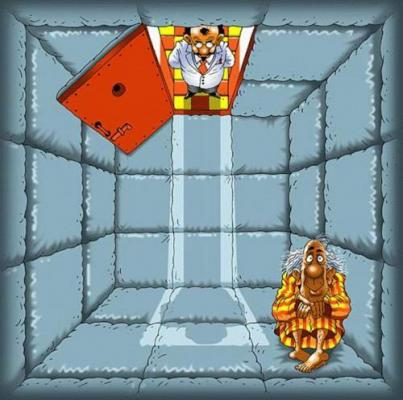##### Share on other sites

Марс в рыбах в квадратуре к юпитеру в тельце :))))))##### Share on other sites

Лилит в Тельце##### Share on other sites

Венера в Стрельце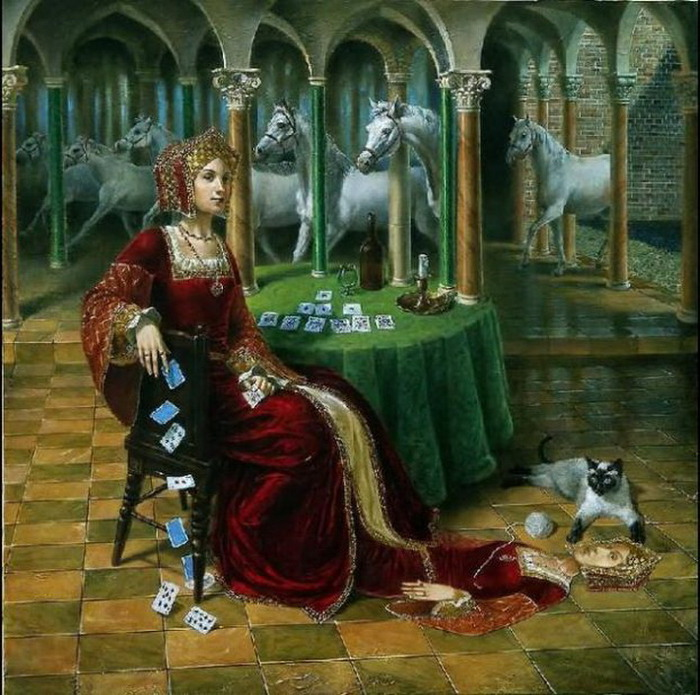)) плинн...будто Венера в Козероге...)) сиамец - доказательство! )) это иховые самые любимишные котейки! )))

смеюсь..)))

##### Share on other sites

Секстиль Венера-Нептун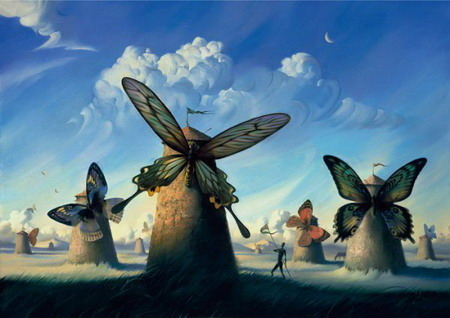##### Share on other sites

Продолжая енотную тему - Марс в близнецах :)))##### Share on other sites

Продолжая енотную тему - Марс в близнецах :)))

Не поверишь! Я только щас подумала:

как бы выглядел мой перводомный близнецовский Марсик!

Ну вот и он! Банзай!!! :)))

##### Share on other sites

Енотная тема - солнце в водолее :))))

Оригинальная конструкция из живых енотов висящих (с какой целью?) на голом осеннем дереве на фоне городских железобетонных пейзажей и вечного неба... непостижимость, короче :)))))##### Share on other sites

Енотная тема - солнце в водолее :))))

Оригинальная конструкция из живых енотов висящих (с какой целью?) на голом осеннем дереве на фоне городских железобетонных пейзажей и вечного неба... непостижимость, короче :))))))))) и чой-та мы там все делаем такой ..тесной группой??))))

группироваться - не свойственно водолеям! ))))

ну, разве то по спецательному заданию от ГЛОАСС, ток если...???))))

а! ясно! )) ето мы там залезли все спецательно, чтобы на фоне железобетонных конструкций смотреться особенно живописно.

Тады - да, тады ето - по-нашему,

по-водолейски! )))

ток все одно, водолеи дурацкими группами на дураццкие деревья в дураццких местах хором не залазиют..)))))))))))

##### Share on other sites

Плутон в скорпе в 8 доме :)))))## Join the conversation

You can post now and register later. If you have an account, sign in now to post with your account.×   Pasted as rich text.   Paste as plain text instead

Only 75 emoji are allowed.